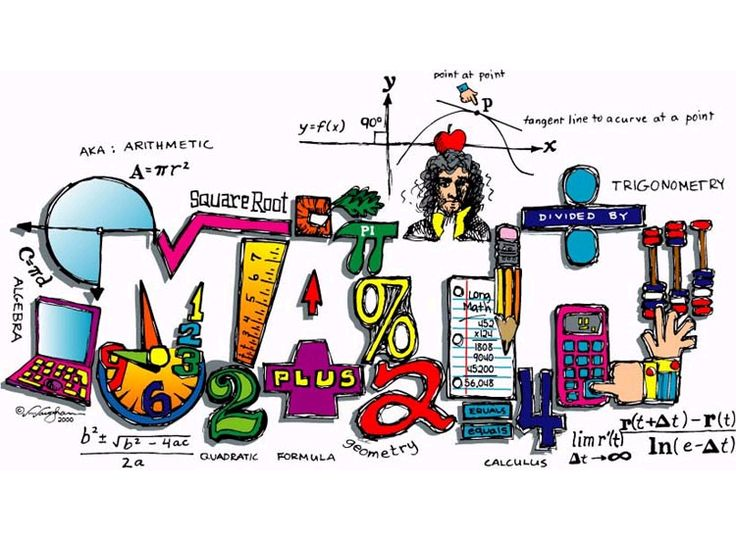•Welcome To Miss Serrano's Page
•

This year I am teaching PreCalulus CP, Algebra III, and Statistics CP.

All of the homework assignments and resources for your course can be found on the google classroom site located to the right.

Assignments can also be found on Genesis.

All announcements for the class will be in google classroom that can also be accessed on the right.

• Course Descriptions

PreCalculus CP:

This college preparatory course covers all the fundamental topics that prepare students for calculus. Emphasis is on problem solving and the study of relations, functions, equation solving, and graphing. The functions studied include polynomial, conics, rational, exponential, logarithmic, trigonometric, and inverse functions. Upon entering this course, students must have a strong working knowledge of the mechanics of Algebra II, and be able to grasp the more theoretical concepts that form the foundation for calculus. Scientific and graphing calculators are required.

Algebra III:

This course is offered as the fourth year course in a four-year sequence that provides students with a modified version of the traditional Algebra I, Geometry, Algebra II, and Advanced Algebra/Trigonometry course sequence. The major part of the course strengthens Algebra skills and concepts. Emphasis is on solving equations and problem solving. Functions studied include polynomial, rational, logarithmic and exponential. Additionally, students start exploration of Trigonometry and the Unit Circle. A graphing calculator is required.

Statistics CP:

This course is an introductory, non-calculus based study of statistics designed as an elective math course for Juniors and Seniors only. Students are introduced to major concepts and tools for collecting, analyzing, and drawing conclusions from data. Students are exposed to four broad conceptual themes: Exploring Data, Planning a Study, Anticipating Patterns, and Statistical Inferences. This course would prepare students for AP Statistics or the college course equivalent. A good working knowledge of Algebra II will be required from the beginning of the course. Graphing calculators are required.